# 2nd PUC Maths Question Bank Chapter 13 Probability Ex 13.1

Students can Download Maths Chapter 13 Probability Ex 13.1 Questions and Answers, Notes Pdf, 2nd PUC Maths Question Bank with Answers helps you to revise the complete Karnataka State Board Syllabus and score more marks in your examinations.

## Karnataka 2nd PUC Maths Question Bank Chapter 13 Probability Ex 13.1

### 2nd PUC Maths Probability NCERT Text Book Questions and Answers Ex 13.1

Question 1.
Given that E and F are events such that P(E) = 0.6, P(F) = 0.3 and P(E∩F) =0.2, find p(E|f) and P(F|E).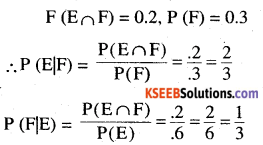Question 2.
Compute P(A|B), if P(B) = 0.5 and P (A∩B) = 0.32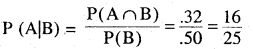Question 3.
If P(A) = 0.8, P(B) = 0.5 and P(B|A) = 0.4 Find.
(i) P(A∩B)
(ii) P(A|B)
(iii) P(A∪B)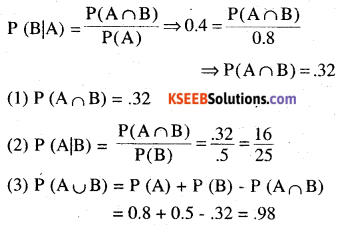Question 4.
Evaluate P(A∪B), if 2P(A) = $$P(B)=\frac { 5 }{ 13 } { and }{ \quad P }({ A }|{ B })=\frac { 2 }{ 5 }$$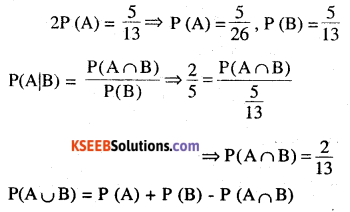Question 5.
$$\text { If } P(A)=\frac{6}{11}, P(B)=\frac{5}{11} \text { and } P(A \cup B)=\frac{7}{11}$$ find
(i) P(A∩B)
(ii) P(A|B)
(iii) P(B|A)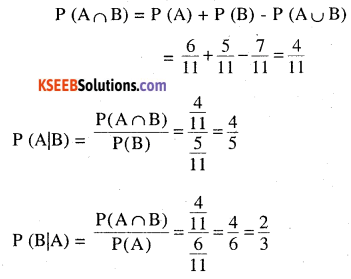Question 6.
A coin is tossed three times, where
(i) E : head on third toss, F : heads on first two tosses
(ii) E : at least two heads, F : at most two heads
(iii) E : at most two tails, F : at least one tail
sample space {HHH, HHT, HTT, TTT, THH, TTH, THT, HTH}Question 7.
Two coins are tossed once, where
(i) E : tail appears on one coin, F : one coin shows head
(ii) E: no tail appears, F: no head appears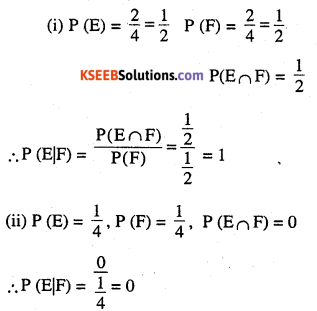Question 8.
A die is thrown three times,
E : 4 appears on the third toss,
F : 6 and 5 appears respectively on first two tosses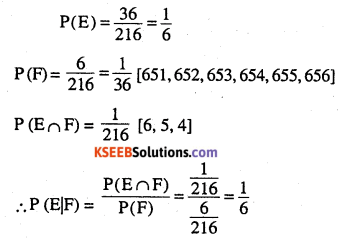Question 9.
Mother, father and son line up at random for a family picture
E : son on one end,
F : father in middle
sample space [MFS, MSF, SMF, SFM, FMS, FSM]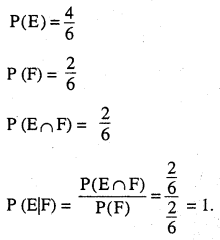Question 10.
A black and a red dice are rolled.
(a) Find the conditional probability of obtaining a sum greater than 9, given that the black die resulted in a 5.
(b) Find the conditional probability of obtaining the sum 8, given that the red die resulted in a number less than 4.
(a) E : Sum gr than 9 = {(4,6), (6,4), (5,5), (6,5), (5,6), (6,6)}
F : Black resulted in 5 = {(5,1), (5,2), (5,3), (5,4),(5,5), (5,6)}
E∩F = sum gr than 9 and black die resulted in 5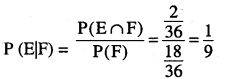Question 11.
A fair die is rolled. Consider events E = {1,3, 5}, F = (2,3} and G = {2, 3,4,5} Find
(i) P (E|F) and P (F|E)
(ii) P (E|G) and P (G|E)
(iii) P ((E∪F)|G) and P (E∩F)|G)Question 12.
Assume that each born child is equally likely to be a girl. If a family has two children, what is the conditional probability that both are girls given that
(i) the youngest is a girl.
(ii) at least one is girl ?
Ans:
E : Both are girls {GG, BB, GB, BG}
F: Youngest is a girl
G: At least one is girl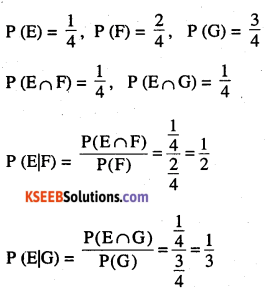Question 13.
An instructor has a question bank  consisting of 300 easy True/False questions, 200 difficult True/False questions, 500 easy multiple choice question and 400 difficult multiple choice questions. If a question is selected at random from the question bank, what is the probability that it will be an easy question given that it is a multiple choice question ?
E : Easy question
F: Multiple choice question Given 300 – E (T|F)
500 – E multiple 200 – D (T|F)
400 – D (multiple)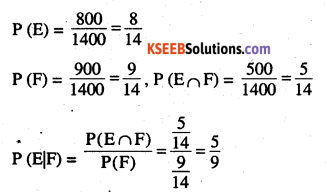Question 14.
Given that the two numbers appearing on throwing two dice are different. Find the probability of the event ‘the sum of numbers on the dice is 4
E : Sum of the number is 4 [(1,3), (3,1), (2,2)]
F : The number appearing are different E∩F different number but sum is 4 throwing two dice are different. Find the probability of the event ‘the sum of numbers on the dice is 4′.
E : Sum of the number is 4 [(1,3), (3,1), (2,2)]
F : The number appearing are different
E∩F : different number but sum is 4
$$P(F)=\frac{30}{36}$$
{except (1,1) , (2,2) , (3,3) ,(4,4) ,(,5,5) ,(6,6)}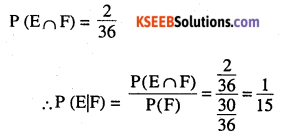Question 15.
Consider the experiment of throwing a die, if a multiple of 3 comes up, throw the 1 die again and if any other number comes, toss a coin. Find the conditional probability of the event ‘the coin shows a tail’, given that ‘at least one die shows a 3’.
{(3,1), (3,2), (3,3), (3,4), (3,5), (3,6), (1,H), (1,T), (2,H), (2,T), (4,T), (4,H), (5,T), (5,H), (6,T),(6,H)}
E: coin shows a tail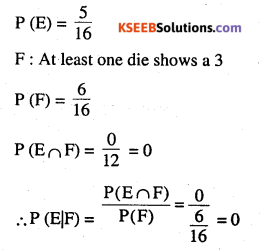∴ Event is called impossible event
In each of the Exercise 16 and 17 choose the correct answer:Question 16.
If P(A) = $$\frac{1}{2}$$,P(B) = 0, then P( A|B) is
(A) 0
(B) $$\frac{1}{2}$$
(C) not defined
(D) 1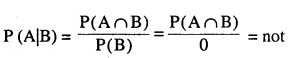defined
Option “C”

Question 17.
If A and B are events such that P (A|B) = P (B|A), then
(A) A⊂B but A≠B
(B) A = B
(C) A∩B =φ
(D) P(A) = P(B)